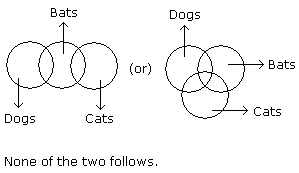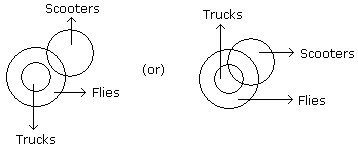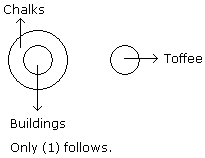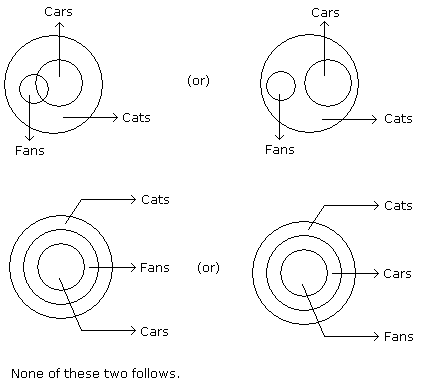# Verbal Reasoning - Syllogism

Exercise : Syllogism - Syllogism 1
Directions to Solve

In each of the following questions two statements are given and these statements are followed by two conclusions numbered (1) and (2). You have to take the given two statements to be true even if they seem to be at variance from commonly known facts. Read the conclusions and then decide which of the given conclusions logically follows from the two given statements, disregarding commonly known facts.

• (A) If only (1) conclusion follows
• (B) If only (2) conclusion follows
• (C) If either (1) or (2) follows
• (D) If neither (1) nor (2) follows and
• (E) If both (1) and (2) follow.

11.

Statements: Some dogs are bats. Some bats are cats.

Conclusions:

1. Some dogs are cats.
2. Some cats are dogs.

Only (1) conclusion follows
Only (2) conclusion follows
Either (1) or (2) follows
Neither (1) nor (2) follows
Both (1) and (2) follow
Explanation:12.

Statements: All the trucks are flies. Some scooters are flies.

Conclusions:

1. All the trucks are scooters.
2. Some scooters are trucks.

Only (1) conclusion follows
Only (2) conclusion follows
Either (1) or (2) follows
Neither (1) nor (2) follows
Both (1) and (2) follow
Explanation:Neither (1) nor (2) follows.

13.

Statements: All buildings are chalks. No chalk is toffee.

Conclusions:

1. No building is toffee
2. All chalks are buildings.

Only (1) conclusion follows
Only (2) conclusion follows
Either (1) or (2) follows
Neither (1) nor (2) follows
Both (1) and (2) follow
Explanation:14.

Statements: All cars are cats. All fans are cats.

Conclusions:

1. All cars are fans.
2. Some fans are cars.

Only (1) conclusion follows
Only (2) conclusion follows
Either (1) or (2) follows
Neither (1) nor (2) follows
Both (1) and (2) follow